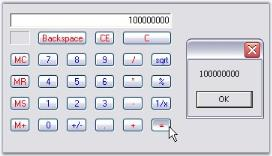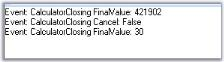# Calculator Events in Windows Forms Calculator

26 Apr 20212 minutes to read

The event for Calculator control and PopupCalculator control are discussed in this section.

## ValueCalculated Event

The ValueCalculated event fires each time the value of the Calculator control is changed. That is, even if you just press any digit, this event will be handled.

The event handler receives an argument of type CalculatorValueCalculatedEventArgs. To get the final result, use LastAction property of the CalculatorValueCalculatedEventArgs in the ValueCalculated event.

We can retrieve the value of the Calculator control after ‘=’ button is pressed using the following code snippet.

``````private void calcctrl_ValueCalculated(object sender,CalculatorValueCalculatedEventArgs arg)
{

// Checks the final answer after '=' is pressed.
if(!arg.ErrorCondition && arg.LastAction == CalcActions.CalcOperatorEquals)
MessageBox.Show(calcctrl.Value.ToString());
}``````
``````Private Sub calcctrl_ValueCalculated(ByVal sender As Object, ByVal arg As CalculatorValueCalculatedEventArgs)
If Not arg.ErrorCondition AndAlso arg.LastAction = CalcActions.CalcOperatorEquals Then

' Checks the final answer after '=' is pressed.
MessageBox.Show(calcctrl.Value.ToString())
End If
End Sub``````## Closing Event

### Closing Event of the PopupCalculator Control

This PopupCalculator closing event will be raised by PopupCalculator when closing after “=” button was clicked. We can implement this event to display the final value of the Calculator control as follows.

``````this.popupCalculator1.Closing += new PopupCalculatorClosingEventHandler(this.HandlePopupCalculatorClosingEvent);
public void HandlePopupCalculatorClosingEvent(object sender, CalculatorClosingEventArgs args)
{

//Event logging
string item = args.FinalValue.ToString();
string eventlogmessage = String.Format("Event: {0} FinalValue: {1}\r\n", "CalculatorClosing", item);
Console.WriteLine(eventlogmessage);
}``````
``````Private Me.popupCalculator1.Closing += New PopupCalculatorClosingEventHandler(Me.HandlePopupCalculatorClosingEvent)
Public Sub HandlePopupCalculatorClosingEvent(ByVal sender As Object, ByVal args As CalculatorClosingEventArgs)

'Event logging
Dim item As String = args.FinalValue.ToString()
Dim eventlogmessage As String = String.Format("Event: {0} FinalValue: {1}" & Constants.vbCrLf, "CalculatorClosing", item)
Console.WriteLine(eventlogmessage)
End Sub``````